Linear Regression

Consider a set of $n$ data points $(x_1,y_1)$, ... , $(x_n,y_n)$. Linear regression uses the data points to predict a linear relationship between $x$ and $y$ (and for the single variable case is equivalent to least-squares fit).

Assume that the relation between $x$ and $y$ is of the form $y=ax+b$. The values of $a$ and $b$ are determined by considering how much that assumed relation misses for the data points, i.e., $(ax_i +b)-y_i$ for the $i^{\rm th}$ data point. To minimize the error, we define a "cost function":

$$C(a,b) =\sum_{i=1}^n ((ax_i +b)-y_i)^2 = ((ax_1 +b)-y_1)^2 + \ldots + ((ax_n +b)-y_n)^2\ .$$

The values of $a$ and $b$ that minimize this function are determined by taking the derivatives and setting equal to zero:

$$0={dC\over db} = \sum_{i=1}^n 2((ax_i +b)-y_i) = 2(a\sum_{i=1}^n x_i + nb -\sum_{i=1}^n y_i ) =2n(a\overline{x}+b-\overline{y})$$$$0={dC\over da} = \sum_{i=1}^n 2((ax_i +b)-y_i)x_i = 2(a\sum_{i=1}^n x_i^2 + b\sum_{i=1}^n x_i - \sum_{i=1}^n y_ix_i)= 2n(a\overline{x^2}+b\overline{x}-\overline{xy})$$

having set $\overline{x}= {1\over n}\sum_{i=1}^n x_i$ (the average value of the $x_i$), $\overline{y}= {1\over n}\sum_{i=1}^n y_i$ (the average value of the $y_i$), and similarly using the additional averages $\overline{xy}={1\over n}\sum_{i=1}^n x_iy_i$ and $\overline{x^2}={1\over n}\sum_{i=1}^n x_i^2$. Then the first equation above becomes

$$b = \overline{y} - a \overline{x}$$

and substituting for $b$ in the second equation gives $0 = a\overline{x^2} + (\overline{y} - a \overline{x})\overline{x} - \overline{xy}$ . Solving for $a$ gives the result

$$a= { \overline{xy} - \overline{x}\,\overline{y} \over \overline{x^2} - \overline{x}^2} = {\overline{(x-\overline{x})}\overline{(y-\overline{y})} \over \overline{(x-\overline{x})^2}}={{\rm Cov}[x,y]\over {\rm Var}[x]}$$

The covariance Cov$[x,y]={1\over n}\sum_{i=1}^n (x_i - \overline{x})(y_i - \overline{y})$ measures the average of how much $x$ and $y$ are both on the same side of their means ($+$ contribution) or on opposite sides ($-$ contribution), so is overall positive when they're correlated, and negative when anti-correlated. The covariance of a random variable with itself is just the ordinary variance: Cov$[x,x]$ = Var$[x]$, measuring the average square of the deviation from the mean.

The two formulae above for $a$ and $b$ are readily calculable for any set of data points $(x_1,y_1)$, ... , $(x_n,y_n)$. Let's generate $n=100$ synthetic data points, with $x$ values between 0 to 10 (recall rand(n) generates n random values between 0 and 1):

In :
n=100
x=10*rand(n)
y=1.5*x + normal(0,2.5,size=n)


The $y$ values in the above are given by multiplying the $x$ values by 1.5 (a linear slope), and then adding noise by pulling 100 independent numbers from a normal distribution (with mean 0 and standard deviation 2.5). The data looks like this:

In :
figure(figsize=(6,5))
plot(x,y,'o');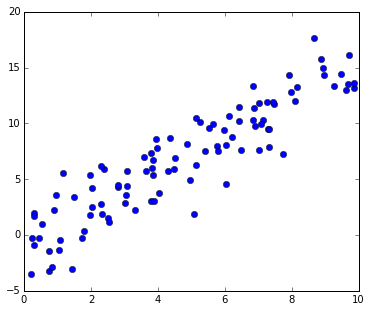Now calculate the best linear fit slope $a$ and $y$-intercept $b$ using the formulae derived above:

In :
xbar=mean(x)
ybar=mean(y)
a=(x.dot(y)/n-xbar*ybar)/(x.dot(x)/n-xbar*xbar)
b=ybar-a*xbar
print a,b

1.45955689947 0.191167623632


The slope is close to the generating value, and together with the above data points looks like this:

In :
figure(figsize=(6,5))
plot(x,y,'o')
xr=arange(11)
plot(xr,a*xr+b,linewidth=3);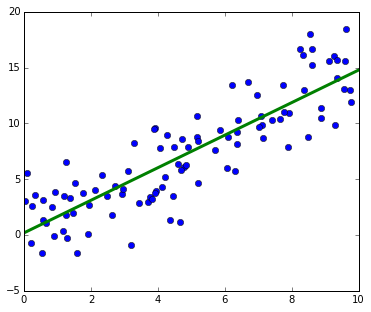These equations for linear regression are commonly enough used that they're already defined as a function linregress in the standard module scipy.stats, which returns the same values for the slope and intercept:

In :
from scipy.stats import linregress
slope, intercept, r_value, p_value, std_err = linregress(x,y)

print 'a =',slope,', b=',intercept,', \xcf\x83=', std_err
print "Pearson's r =",r_value, ', p-value=',p_value

a = 1.45955689947 , b= 0.191167623632 , σ= 0.0858274566043
Pearson's r = 0.864232048349 , p-value= 5.35418781675e-31


linregress() returns as well three other quantities:

First, the std_err is a measure of the stdev of the slope estimation (under the assumption that the errors $(ax_i+b)−y_i$ are normally distributed), $\sigma= \sqrt{{1\over n-2}C(a,b) \big/ n{\rm Var}[x]}$ :

In :
sqrt((1./(n-2))*(a*x+b -y).dot(a*x+b -y) / (x-xbar).dot(x-xbar))

Out:
0.085827456604302785

The other two quantities returned by scipy.stats.linregress() are known as the Pearson's r correlation, and its associated p-value. Pearson's r correlation (or $\rho$, for Greek rho) between two variables is related to the above covariance, Cov[x,y], except normalized so that it ranges between a minimum of -1 (for completely anticorrelated) to a maximum of +1 (for completely correlated): $$\rho_{x,y}={{\rm Cov}[x,y]\over \sigma[x]\sigma[y]} ={E[(x-E[x])(y-E[y])]\over \sigma[x]\sigma[y]}\ .$$ Dividing by $\sigma[x]\sigma[y]$ keeps it symmetric in $x$ and $y$, and also means that when $y=x$ (maximally correlated), it becomes ${\rm Cov}[x,x]/\sigma[x]\sigma[x]={\rm Var}[x]/{\rm Var}[x]=1$, and similarly is equal to -1 if $y=-x$ (since from the definition, ${\rm Cov}[x,-x]=-{\rm Var}[x]$). This also agrees with the output of linregress():

In :
(x.dot(y)/n-xbar*ybar)/sqrt((x.dot(x)/n-xbar*xbar)* (y.dot(y)/n-ybar*ybar))

Out:
0.86423204834868328

Pearson's r measures something slightly different from the slope. If two variables have data points falling on the same straight line then it will be 1 (or -1) independent of the slope, and it will be between -1 and 1 if there are deviations from the straight line fit, again independent of the slope, as illustrated in this figure (from Wikipedia):

In :
from urllib2 import urlopen
from IPython.display import Image

Out: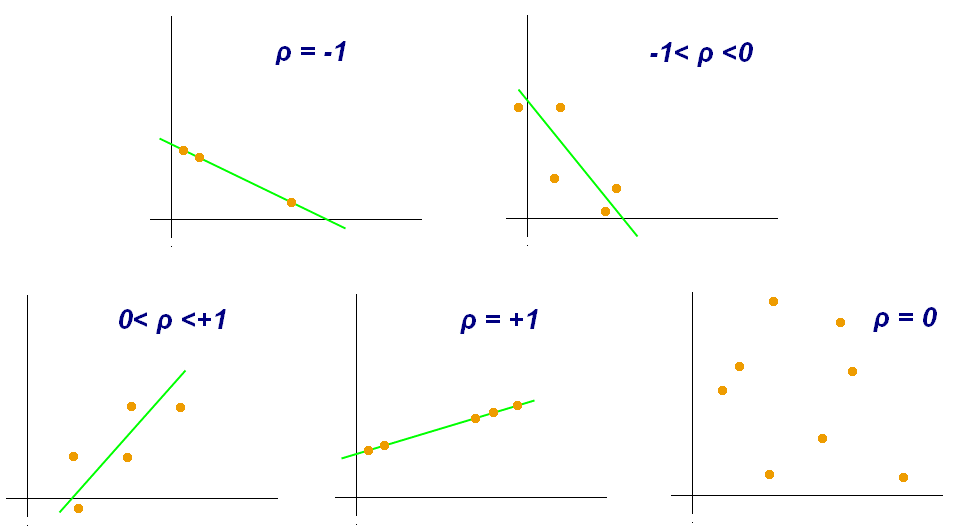The Pearson r is also used sufficiently that it has its own dedicated implementation as scipy.stats.pearsonr(), giving the same values:

In :
from scipy.stats import pearsonr
pearsonr(x,y)

Out:
(0.86423204834868272, 5.3541878167471908e-31)

The p_value returned by linregress() (or pearsonr()) is a measure of how significant it is that the correlation is non-zero. One way of assessing this is to randomly permit the y values and to recalculate correlation:

In :
import random
yr=random.sample(y,100)
figure(figsize=(6,5))
plot(x,yr,'o');
print pearsonr(x,yr)

(-0.032637855742317069, 0.74717917356035102)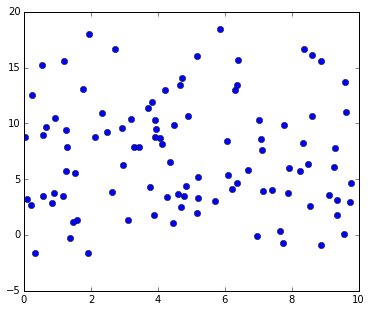Now the correlation is just .04, with a large probability (p-value) that the small non-zero value is a statistical fluctuation. One way to view the p-value for the original data is as the probability that one would get that large a correlation coefficient by chance if the variables were actually uncorrelated. Equivalently, that means the probability that the correlation coefficient would be as large as measured after a random permutation of the y values.

Logistic Regression

The last thing discussed in lecture was a modification of the regression scheme when trying to predict a binary outcome rather than a linear relation. This makes use of the so-called "logistic function":

$${\rm logit}(x) = {1\over 1+{\rm e}^{-x}}$$

For large positive values of the argument $x$, ${\rm e}^{-x}$ becomes very small so the function evaluates to 1, whereas for large negative values of $x$, ${\rm e}^{-x}$ becomes very large and the function evaluates to zero. The value for $x=0$ is logit(0)=.5, and that marks the middle of the transition region between 0 and 1.

$x$ can be considered to be any linear combination of feature values $f_i$ being used to make the binary prediction, $x=\alpha_1 f_1 + \ldots + \alpha_n f_n + \beta$, where the weights $\alpha_i$ and $\beta$ are determined by some training procedure (to provide the best fit to labelled training data). Intermediate values of the logit function can be interpreted as the probability of the label being 1 for those values of the features.

For a single feature $x=\alpha f_1+\beta$, the logit is equal to .5 for $f_1=-\beta/\alpha$, and the width of the transition region is proportional to $1/\alpha$:

In :
def logit(x): return 1/(1+exp(-x))

In :
f1=arange(-10,10,.1)
plot(f1,logit(f1),label='logit($f_1$)')
plot(f1,logit(10*f1-30),label='logit($10f_1-30$)')
yticks(arange(0,1.1,.1))
grid('on')
legend(loc='upper left');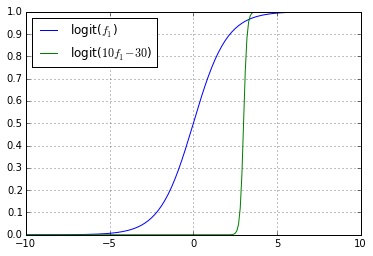In [ ]: